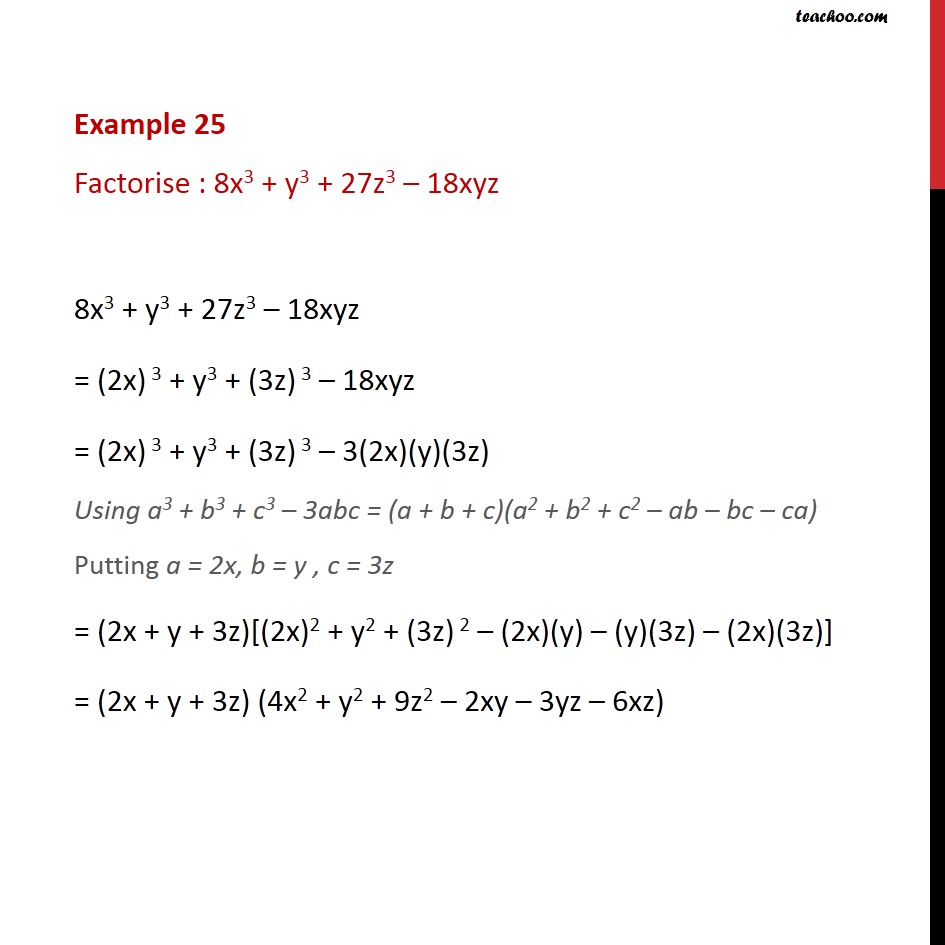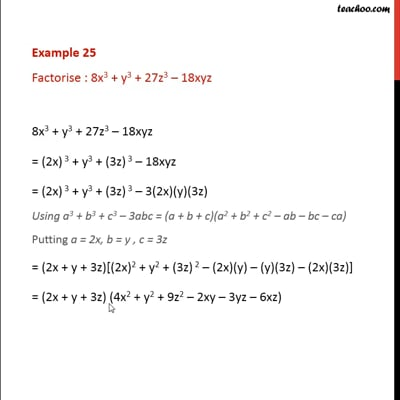Identity VIII

Chapter 2 Class 9 Polynomials (Term 2)
Concept wiseThis video is only available for Teachoo black users

### Transcript

Example 25 Factorise : 8x3 + y3 + 27z3 – 18xyz 8x3 + y3 + 27z3 – 18xyz = (2x) 3 + y3 + (3z) 3 – 18xyz = (2x) 3 + y3 + (3z) 3 – 3(2x)(y)(3z) Using a3 + b3 + c3 – 3abc = (a + b + c)(a2 + b2 + c2 – ab – bc – ca) Putting a = 2x, b = y , c = 3z = (2x + y + 3z)[(2x)2 + y2 + (3z) 2 – (2x)(y) – (y)(3z) – (2x)(3z)] = (2x + y + 3z) (4x2 + y2 + 9z2 – 2xy – 3yz – 6xz)